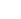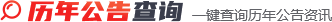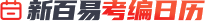2023年02月06日~02月12日MORE+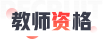MORE+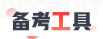MORE+MORE+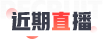MORE+MORE+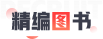MORE+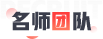MORE+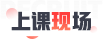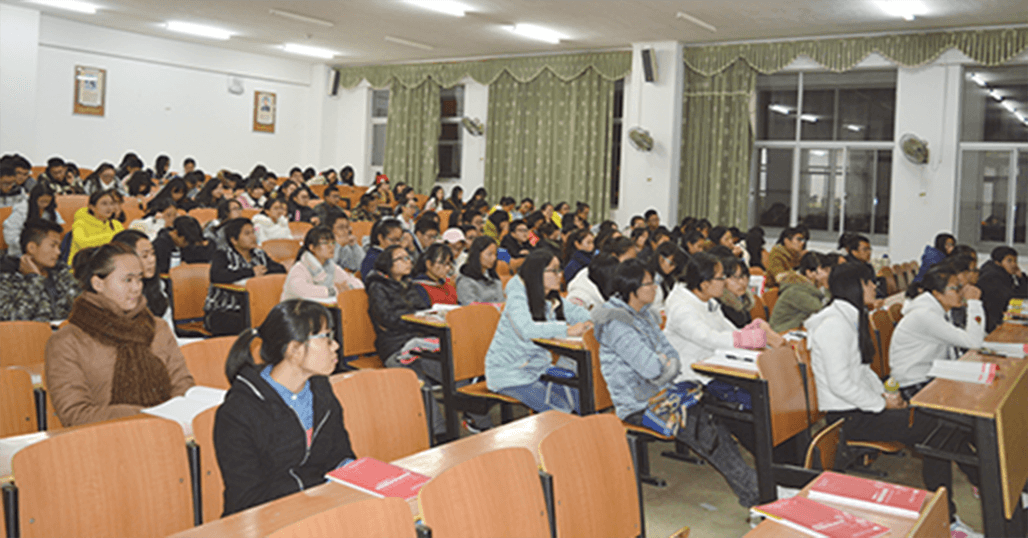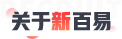MORE+

700 万+23600 课时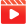30000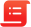89.5 %以上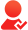MORE+© 2023 Baidu - GS(2021)6026号 - 甲测资字11111342 - 京ICP证030173号 - Data © 百度智图
#
R
G
B
H
S
B
OK
#
R
G
B
H
S
B
OK
#
R
G
B
H
S
B
OK
#
R
G
B
H
S
B
OK
#
R
G
B
H
S
B
OK
#
R
G
B
H
S
B
OK
#
R
G
B
H
S
B
OK
#
R
G
B
H
S
B
OK
#
R
G
B
H
S
B
OK
#
R
G
B
H
S
B
OK
#
R
G
B
H
S
B
OK
#
R
G
B
H
S
B
OK
#
R
G
B
H
S
B
OK
#
R
G
B
H
S
B
OK
#
R
G
B
H
S
B
OK
#
R
G
B
H
S
B
OK
#
R
G
B
H
S
B
OK
#
R
G
B
H
S
B
OK
#
R
G
B
H
S
B
OK
#
R
G
B
H
S
B
OK
#
R
G
B
H
S
B
OK
#
R
G
B
H
S
B
OK
#
R
G
B
H
S
B
OK
#
R
G
B
H
S
B
OK
#
R
G
B
H
S
B
OK
#
R
G
B
H
S
B
OK
#
R
G
B
H
S
B
OK
#
R
G
B
H
S
B
OK
#
R
G
B
H
S
B
OK
#
R
G
B
H
S
B
OK
#
R
G
B
H
S
B
OK
#
R
G
B
H
S
B
OK
#
R
G
B
H
S
B
OK
#
R
G
B
H
S
B
OK
#
R
G
B
H
S
B
OK
#
R
G
B
H
S
B
OK
#
R
G
B
H
S
B
OK
#
R
G
B
H
S
B
OK